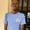# 5 Real-Life Uses for the JavaScript reduce() MethodIn this article, we'll take a look at some handy uses for the JavaScript array reduce method. I'll show you five practical uses, including finding the sum of an array.

## What Is the reduce() Method?

The JavaScript array reduce method is a built-in iteration method used for performing an accumulative action on an array:

The method accepts a callback function that's called once for every item in the array. Every callback function call must always return an accumulated value.

The second argument passed to reduce() is an optional initial value which is used as the accumulator on the first iteration. If the second argument is omitted, the first item in the array will be used as the initial value instead.

The JavaScript reduce() method is very versatile, with some really handy use cases, even though it is widely considered to be the most confusing of all JavaScript iterators.

Let's take a look at some use cases for the JavaScript reduce() method.

## 1. Summing an Array Using reduce()

The easiest and perhaps most popular use of the JavaScript reduce method is to get the sum total of a list of numbers.

For example, here's the code for summing together the total points using reduce:

On every iteration, we simply added the current teams' points to the previously accumulated points, and then we returned that as the accumulated value for the next iteration.

Another very similar use for JavaScript reduce() is to get the total count of items within an array collection, like getting the team count:

Of course, it's better to use the count property to get the total count of an array, but this example shows how you can count using reduce() as well.

## 2. Counting Occurrences of Items in an Array Using reduce()

Another simple use for JavaScript reduce() is to obtain the number of times each item appears inside an array collection.

For example, here is the code for getting the total number of occurrences for each fruit in an array of fruits:

Note that at each step of the iteration, the accumulator will be a hash map (i.e. a JavaScript object) with a key for each fruit in the array so far, and the values will be the number of times each fruit has occurred. This is the expression where we update the accumulator:

Let's break this down a little. First, using the spread operator, ...acc copies over all the existing values from the accumulator.

Then, [currFruit]: sets the value for the current fruit in the array. For example, on the very first iteration over the fruits collection, this will create the Banana property. acc[currFruit] || 0 retrieves the value for that fruit from the previous version of the accumulator. If the fruit doesn't exist in the accumulator, it will start with a value of zero.

Finally, we add 1 to the value of that fruit and return the new accumulator.

## 3. Converting Between Types Using reduce()

You can also reduce an array to any other data type.

For example, suppose we want to get just the names of each student from an array of objects. We can reduce the array from an array of objects to an array of string values, like so:

In the code above, we accessed just the names on each iteration, and passed each name into the accumulated array using the spread operator. Since an array literal was set as the initial value, it was used as the wrapper for the final values.

Normally, we'd use map() for this use case, but I wanted to show you how we can do almost anything with reduce(). In many ways, the JavaScript reduce method is like the map method, but on steroids.

In the following example, we will reduce a students array to a single object:

Here, we restructured our data collection from an array of objects into a single object.

## 4. Getting Max and Min Values From an Array Using reduce()

You can easily obtain the maximum and minimum numerical values from an array in a few lines of code, using JavaScript reduce().

For example, we can obtain the maximum score from a list of scores, like so:

In the code above, at each iteration step we check if the current score value is greater than the accumulated value, or if the accumulator is null. If so, we update the accumulator with the new high score.

In other words, we just return the highest score so far at each step.

Conversely, in the following example, we'll be obtaining the minimum value instead:

The only difference between this and that of max is the change in operator—from greater than to less than.

## 5. Flatten a List of Arrays Using reduce()

The reduce() method has another handy use: flattening nested arrays.

For example, we can flatten a list of arrays into a single array, like so:

In this snippet, we just concatenate each array into the accumulator, merging them into a single array.

## Conclusion

The JavaScript reduce() is an in-built array method used to perform an accumulative action on an array.

The method has a wide array of uses, from summing up values to flattening array lists.

Though the method is very versatile and can be used in many cases, it is important to consider other specialized methods for specific tasks: like using find() to find an item or map() to transform each of the elements in an array.